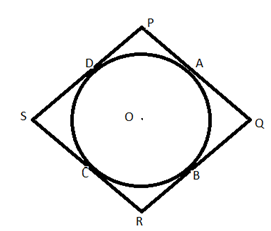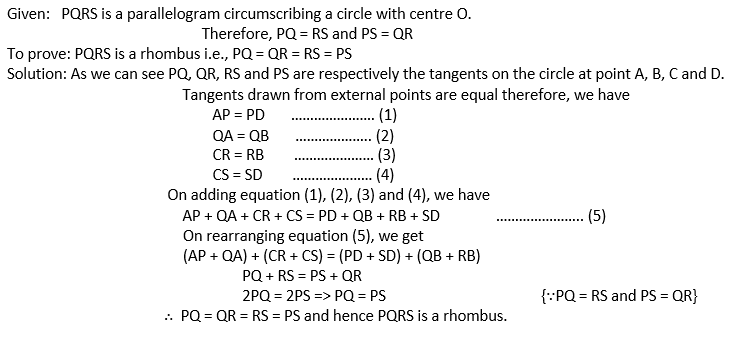# Chapter 10 Circles

At Saralstudy, we are providing you with the solution of Class 10 Mathematics Circles according to the latest NCERT (CBSE) Book guidelines prepared by expert teachers. Here we are trying to give you a detailed answer to the questions of the entire topic of this chapter so that you can get more marks in your examinations by preparing the answers based on this lesson. We are trying our best to give you detailed answers to all the questions of all the topics of Class 10th mathematics Circles so that you can prepare for the exam according to your own pace and your speed.

•  Q1 How many tangents can a circle have? Ans: Circle is the locus of points equidistant from a given point, which is the centre of the circle. And, tangent is the line which intersects a circle at one point only. On these points which touches at only one point. Hence, a circle can have infinite tangents. Q2 Fill in the blanks : (i) A tangent to a circle intersects it in                  point (s). (ii) A line intersecting a circle in two points is called a                  . (iii) A circle can have                       parallel tangents at the most. (iv) The common point of a tangent to a circle and the circle is called                   . Ans: A tangent to a circle intersect it in one point. A line intersecting a circle in two points is called a secant. A circle can have two parallel tangent s at the most. The common point of a tangent of a circle and the circle is called point of contact. Q3 A tangent PQ at a point P of a circle of radius 5 cm meets a line through the centre O at a point Q so that OQ = 12 cm. Length PQ is: Ans: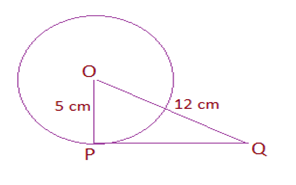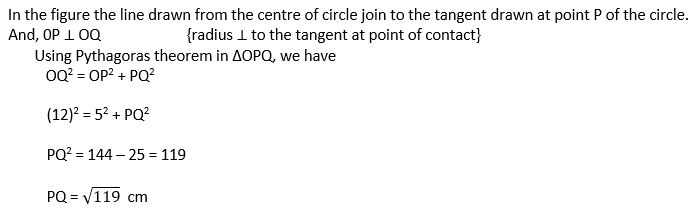Q4 Draw a circle and two lines parallel to a given line such that one is a tangent and the other, a secant to the circle. Ans: In the given figure , AB and BC are two parallel lines. The line segment BC is the tangent at point x while AB is the secant to the circle.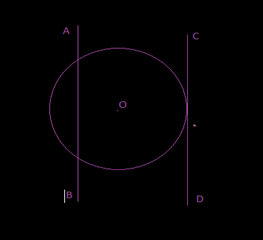•  Q1 From a point Q, the length of the tangent to a circle is 24 cm and the distance of Q from the centre is 25 cm. The radius of the circle is (A) 7 cm (B) 12 cm (C) 15 cm (D) 24.5 cm Ans: Diagram drawn according to the question. Here OP is the radius of the circle.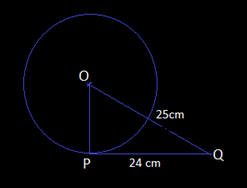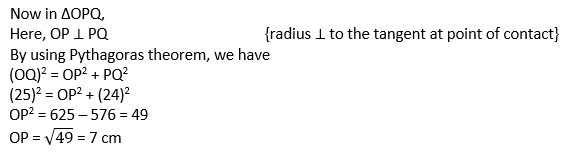Q2 In Fig. 10.11, if TP and TQ are the two tangents to a circle with centre O so that ∠ POQ = 110°, then ∠ PTQ is equal to (A) 60° (B) 70° (C) 80° (D) 90° Ans: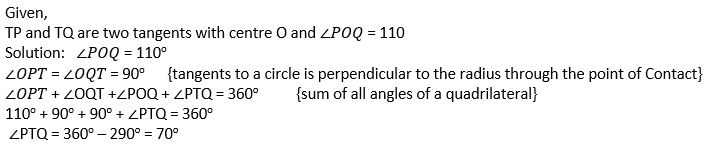Q3 If tangents PA and PB from a point P to a circle with centre O are inclined to each other at angle of 80°, then ∠ POA is equal to (A) 50° (B) 60° (C) 70° (D) 80° Ans: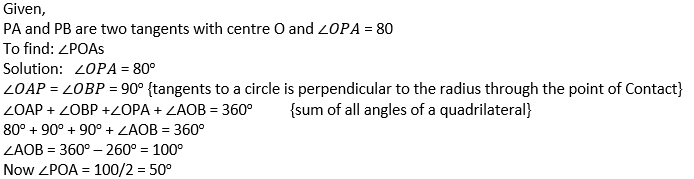Q4 Prove that the tangents drawn at the ends of a diameter of a circle are parallel. Ans: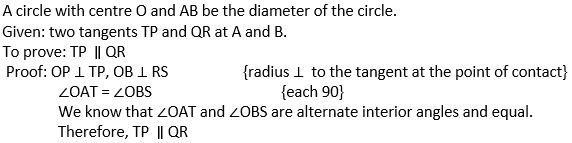Q5 Prove that the perpendicular at the point of contact to the tangent to a circle passes through the centre. Ans: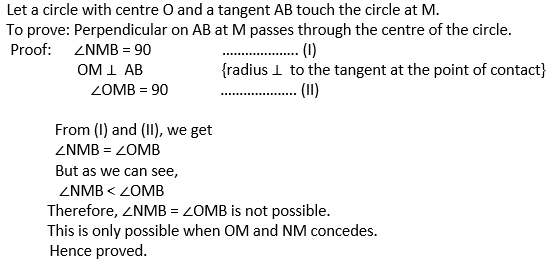Q6 The length of a tangent from a point A at distance 5 cm from the centre of the circle is 4 cm. Find the radius of the circle. Ans: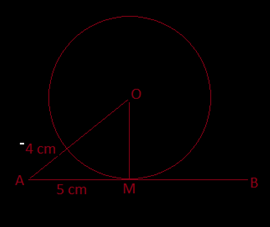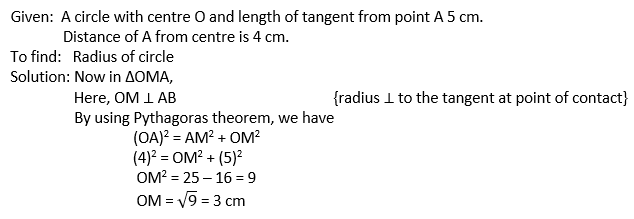Q7 Two concentric circles are of radii 5 cm and 3 cm. Find the length of the chord of the larger circle which touches the smaller circle. Ans: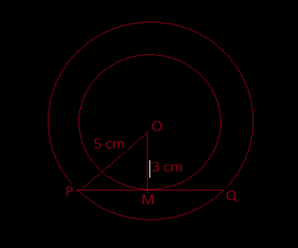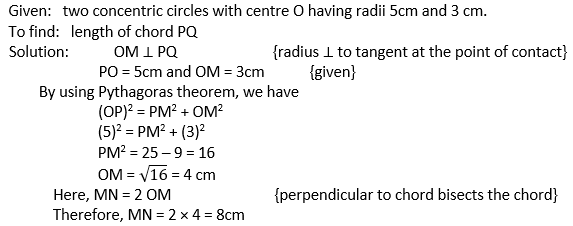Q8 A quadrilateral ABCD is drawn to circumscribe a circle (see Fig. 10.12). Prove that AB + CD = AD + BC Ans: Given :  A quadrilateral ABCD which circumscribe a circle . To prove: AB + CD = AD + BC Proof: As we know tangents drawn from external point are equal. Therefore, we have DR = DS                        …………….. (1) AP = AS                        ……………… (2) PB = BQ                        ……………… (3) CR = CQ                       ………………. (4) Adding equation (1), (2), (3) and (4), we get   DR + AP + PB + CQ = DS + AS + BQ + CR                AB + CD = AD + BC   Hence proved. Q9 In Fig. 10.13, XY and X′Y′ are two parallel tangents to a circle with centre O and another tangent AB with point of contact C intersecting XY at A and X′Y′ at B. Prove that ∠ AOB = 90°. Ans: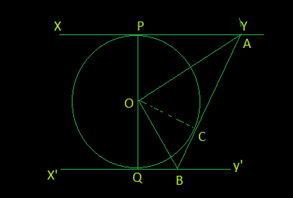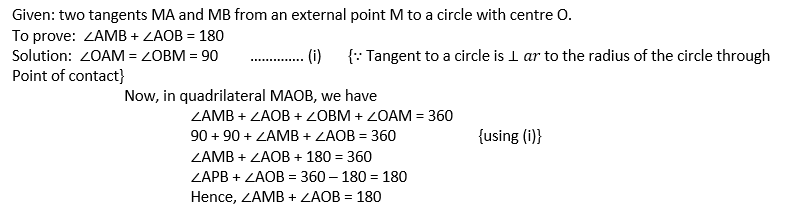Q11 Prove that the parallelogram circumscribing a circle is a rhombus. Ans: